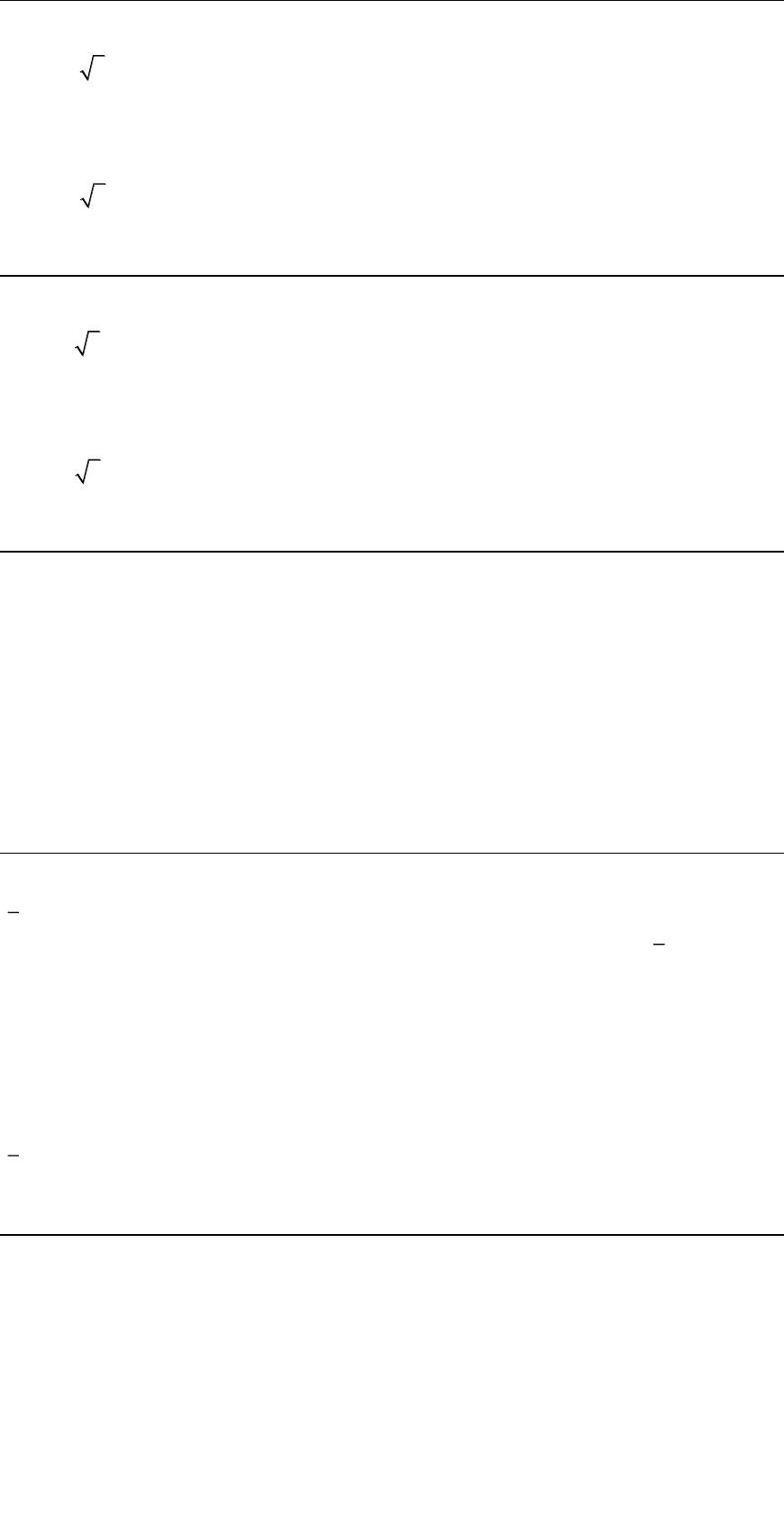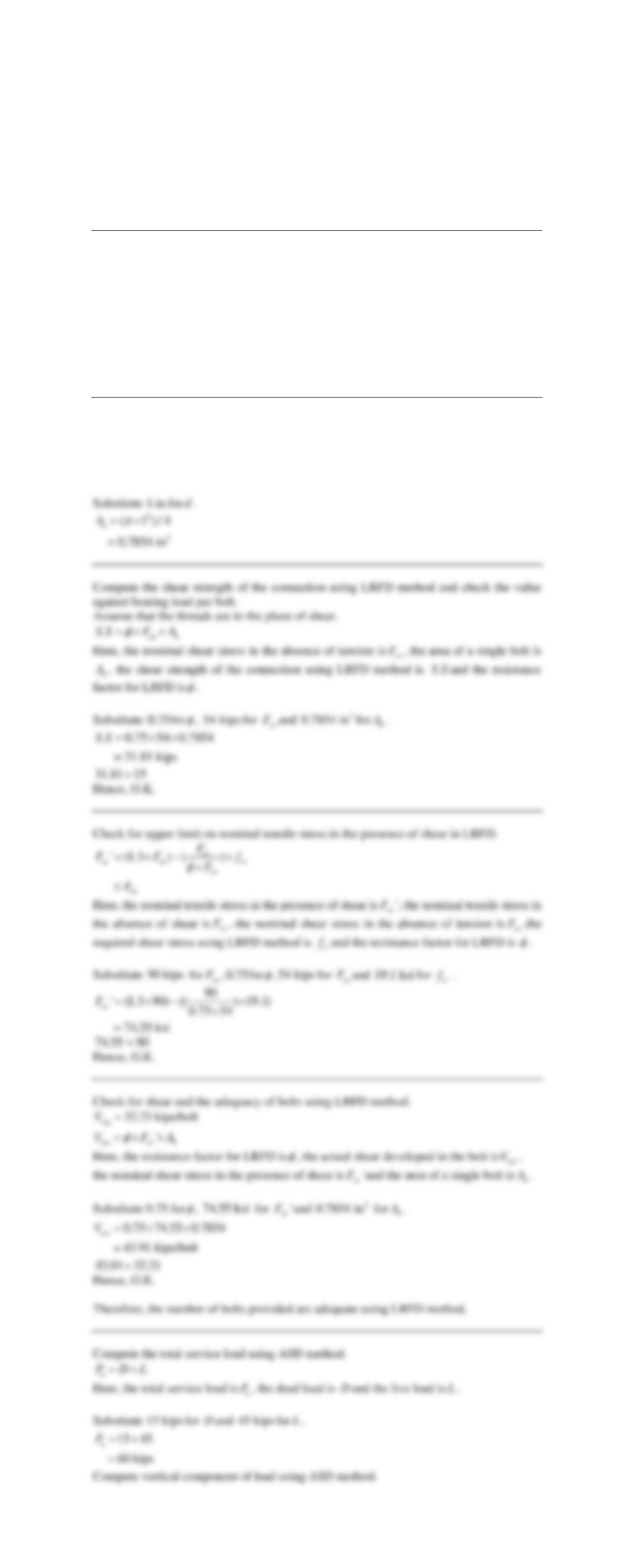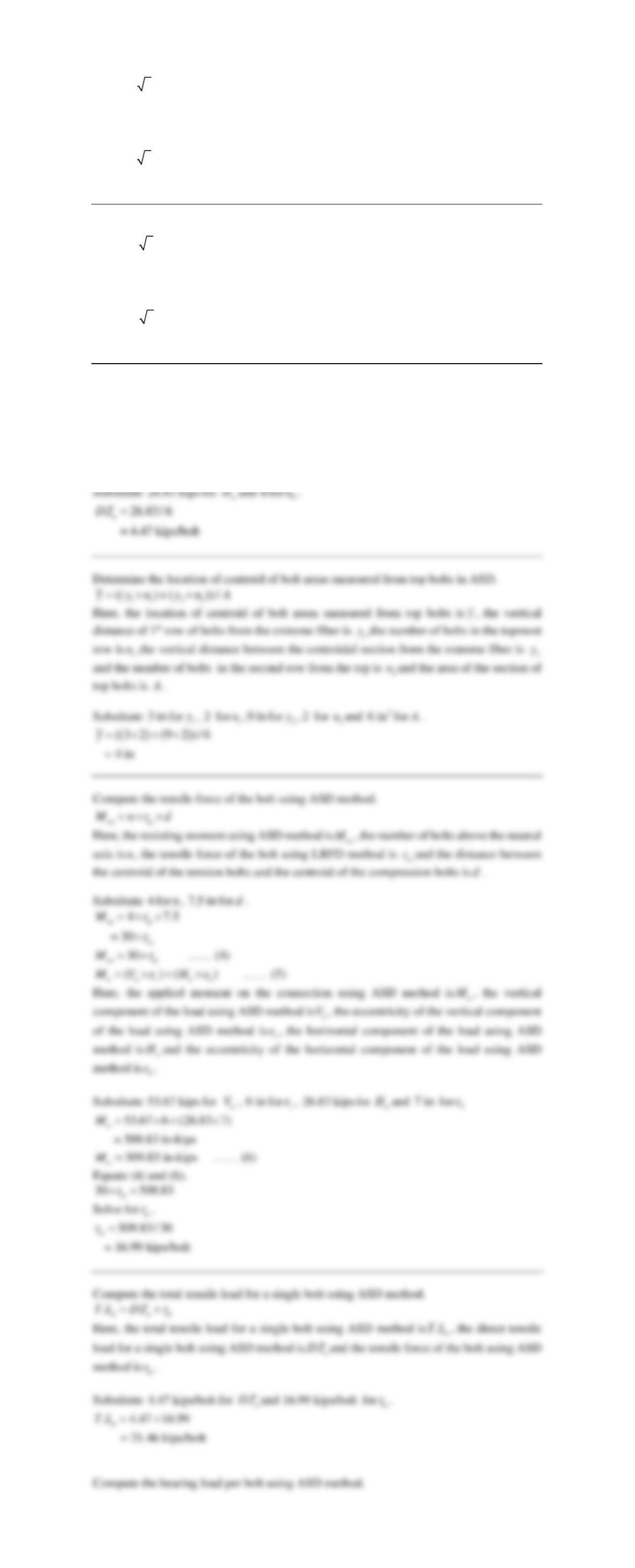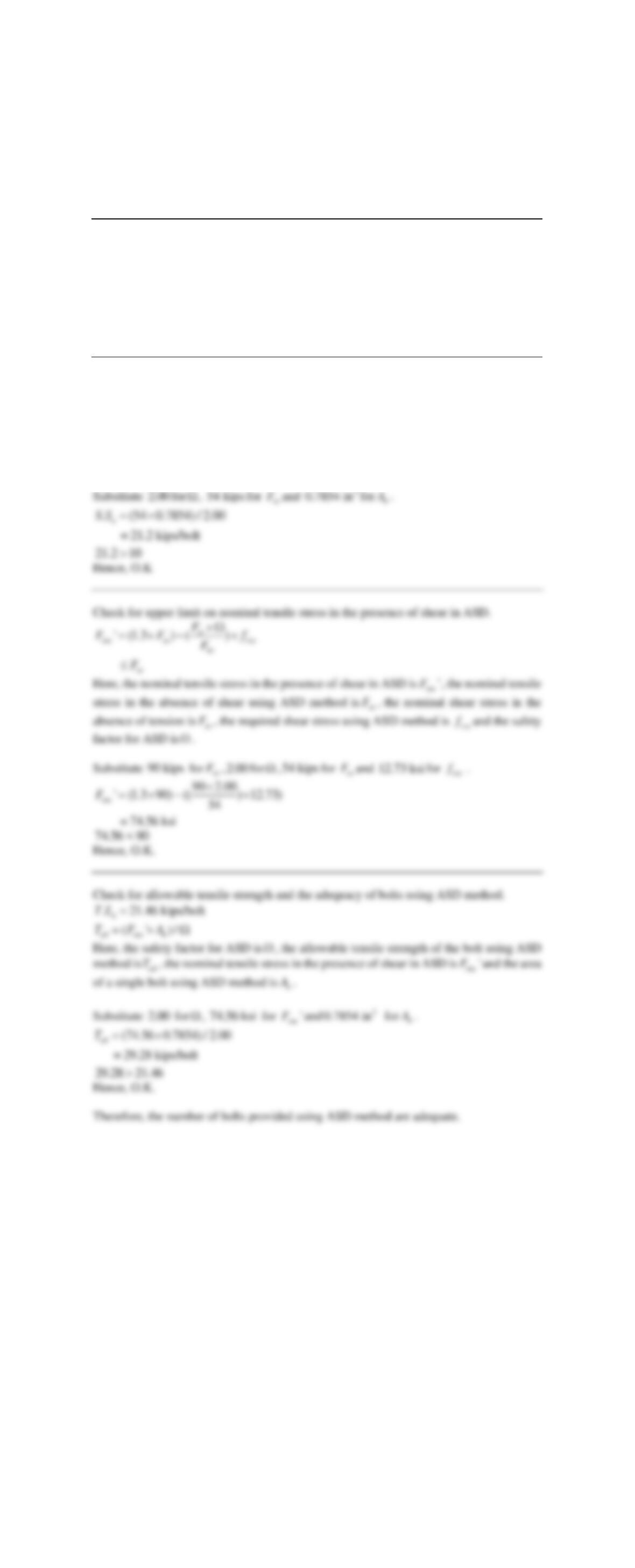Type
Essay
Pages
4 pages
Word Count
1789 words
School
603103
Course Code
603103

# 603103

September 8, 2020Compute the factored load using LRFD method.
(1.2 ) (1.6 )
u
P D L=  +
D
L
u
P
.
Substitute
15 kips
for
D
and
45 kips
for
L
.
Compute the vertical component of factored load using LRFD method.
(2 / 5)
uu
VP=
u
P
and the vertical component of factored load is
u
V
.
Substitute
90 kips
for
u
P
.
(2 / 5) 90
= 80.5 kips
u
V=
Compute the horizontal component of factored load using LRFD method.
(1/ 5)
uu
TP=
u
P
and the horizontal component of factored load is
u
T
.
Substitute
90 kips
for
u
P
.
(1/ 5) 90
= 40.25 kips
u
T=
Calculate the direct tensile load for a single bolt using LRFD method.
/
b u b
V T n=
Here, the direct tensile load for a single bolt using LRFD method is
b
V
, the horizontal
u
T
and the number of bolts in the connection is
b
n
.
Substitute
40.25 kips
for
u
T
and
6
for
b
n
.
40.25 / 6
= 6.71 kips/bolt
b
V=
Determine the location of centroid of bolt areas measured from top bolts in LRFD.
1 1 2 2
(( ) ( ))/y y n y n A=  +
Here, the location of centroid of bolt areas measured from top bolts is
y
, the vertical
distance of 1st row of bolts from the extreme fiber is
1
y
,the number of bolts in the topmost
row is
1
n
,the vertical distance between the centroidal section from the extreme fiber is
2
y
and the number of bolts in the second row from the top is
2
n
and the area of the section of
top bolts is
A
.
Substitute
3 in
for
1
y
,
2
for
1
n
,
9 in
for
2
y
,
2
for
2
n
and
2
6 in
for
A
.
((3 2) (9 2)) / 6
= 4 in
y= + 
Compute the tensile force of the bolt using LRFD method.
t
M n r d=  
Here, the resisting moment is
M
, the number of bolts above the neutral axis is
n
, the tensile
force of the bolt using LRFD method is
t
r
and the distance between the centroid of the
tension bolts and the centroid of the compression bolts is
d
.
Substitute
4
for
n
,
7.5 in
for
d
.
4 7.5
= 30
t
t
Mr
r
=  
30 t
Mr=
…… (1)
( ) ( )
u u v u h
M V e T e=  +
…… (2)
Here, the applied moment on the connection is
u
M
, the vertical component of the factored
u
V
and the eccentricity of the vertical component of the factored load is
v
e
, the
horizontal component of the factored load is
u
T
and the eccentricity of the horizontal
component of the factored load is
h
e
.
Substitute
80.5 kips
for
u
V
,
6 in
for
v
e
,
40.25 kips
for
u
T
and
7 in
for
h
e
80.5 6 (40.25 7)
= 764.75 in-kips
u
M=  +
= 764.75 in-kips
u
M
…… (3)
Equate (1) and (3).
30 764.75
t
r=
Solve for
t
r
.
764.75/ 30
= 25.5 kips/bolt
t
r=
Compute the total tensile load per bolt using LRFD method..bt
T L V r=+
Here, the direct tensile load for a single bolt using LRFD method is
b
V